shell运算符_shell运算符号 - CSDN

• Bash 支持很多运算符，其中包括算数运算符、关系运算符、布尔运算符、字符串运算符和文件测试运算符
目录
文章目录目录概述1. 算数运算符2. 关系（比较）运算符3. 逻辑运算符4. 字符串运算符5. 文件测试运算符
概述
Bash 支持很多运算符，其中包括：
 - 算数运算符
- 关系（比较）运算符
- 逻辑运算符
- 字符串运算符
- 文件测试运算符

1. 算数运算符
a=10
b=20


算术运算符
说明
举例

+
加法
expr $a +$b 结果为30

-
减法
expr $a -$b 结果为-10

*
乘法
expr $a \*$b 结果为200

/
除法
expr $b /$2 结果为2

%
取余
expr $b %$a 结果为0

=
赋值
a=$b 把变量b的值赋值给变量a == 相等。判断两个数字是否相等，相等则返回true [$a == $b ] 返回true != 不相等。判断两个数字是否相等，不相等则返回true [$a != $b ] 返回true 其实原生bash不支持简单的数学运算，但是可以通过其他命令来实现， 例如: awk 和 expr命令进行处理计算之后才可以得出结果，expr 最常用。 expr 是一款表达式计算工具，使用它能完成表达式的求值操作。 # 以下几点需要注意： 1.表达式和运算符之间要有空格。 例如： 5+10 是不对的，必须写成 5 + 10，这与我们熟悉的大多数编程语言不一样。 2.完整的表达式要被   包含，注意这个字符不是常用的单引号，在 Esc 键下边。 3.乘号(*)前边必须加反斜杠(\)进行转义才能实现乘法运算。  举例说明： #!/bin/bash #created by yuki on 20180723 #this script is used for doing ...... a=10 b=20 result=expr$a + $b echo "a + b =$result"

result=expr $a -$b
echo "a - b = $result" result=expr$a \* $b echo "a * b =$result"

result=expr $b /$a
echo " b / a = $result" result=expr$b % $a echo "b % a =$result"

运行结果：
a + b = 30
a - b = -10
a * b = 200
b / a = 2
b % a = 0

2. 关系（比较）运算符
a=5
b=10

关系运算符包括：

在[]中使用的比较符
在(()和[[]]}中使用的比较符
说明
举例

-eq
==
equal的缩写，表相等
[ $a -eq$b ] 返回 false

-ne
!=
not equal的缩写，表不相等
[ $a -nq$b ] 返回 true

-gt
>
greater thanl的缩写，表大于
[ $a -gt$b ] 返回 false

-ge
>=
greater equal的缩写，表大于等于
[ $a -ge$b ] 返回 false

-lt
<
greater equal的缩写，表小于
[ $a -lt$b ] 返回 true

-le
<=
greater equal的缩写，表小于等于
[ $a -lq$b ] 返回 true

提示：
1. "<"符号意思是小于，要麽：if [[ "$a" < "$b" ]] 是错误的，要麽：if [ "$a" \< "$b" ] （即在单[]中需要转义，因为shell也用 <和>表示重定向）。
2. ">"符号意思是大于，要麽：if [[ "$a" > "$b" ]] 是错误的，要麽：if [ "$a" \> "$b" ] （即在单[]中需要转义，因为shell也用 <和>表示重定向）。
3. 关系运算符只支持数字（整数），不支持字符串，除非字符串的值是数字（整数）。
4. 条件表达式要放在方括号之间，并且要有空格，例如 [[$a==$b]] 是错误的，必须写成 [[ $a ==$b ]]。

举例说明：
#!/bin/bash

#created by yuki on 20180723
#this script is used for doing ......

a=5
b=10

if [ $a -eq$b ]
then
echo "eq 关系运算结果: a等于b"
else
echo "eq 关系运算结果: a不等于b"
fi

if [ $a -ne$b ]
then
echo "ne 关系运算结果: a不等于b"
else
echo "ne 关系运算结果: a等于b"
fi

if [ $a -gt$b ]
then
echo "gt 关系运算结果: a大于b"
else
echo "gt 关系运算结果: a小于等于b"
fi

if [ $a -lt$b ]
then
echo "lt 关系运算结果：a小于b"
else
echo "lt 关系运算结果：a大于等于b"
fi

if [ $a -ge$b ]
then
echo "ge 关系运算结果：a大于等于b"
else
echo "ge 关系运算结果：a小于b"
fi

if [ $a -le$b ]
then
echo "le 关系运算结果：a小于等于b"
else
echo "le 关系运算结果：a大于b"
fi

运行结果：
eq 关系运算结果: a不等于b
ne 关系运算结果: a不等于b
gt 关系运算结果: a小于等于b
lt 关系运算结果：a小于b
ge 关系运算结果：a小于b
le 关系运算结果：a小于等于b

3. 逻辑运算符

在[ ]中使用的比较符
在(()和[[]]}中使用的比较符
说明
举例

赋值
a=10
b=25

-a
&&
与运算，两个表达式都为true,才返回true
[ $a -lt 20 -a$b -gt 20 ] 返回true

-o
||
或运算，有一个表达式都为true,则返回true
[ $a -lt 20 -o$b -gt 100 ] 返回true

!
!
非运算，表达式为true,则返回false
[ ! false ] 返回true

小结：逻辑操作符使用总结
[ ]中用 -a,-o, !
[[ ]]中用 &&,||, !
test 用法和 [ ]相同

举例说明：
#!/bin/bash

#created by yuki on 20180723
#this script is used for doing ......

a=5
b=10

if [ $a !=$b ]
then
echo "!= 逻辑非运算结果：a不等于b"
else
echo "!=逻辑非运算结果： a等于b"
fi

if [ $a -lt 50 -a$b -gt 8 ]
then
echo "and 逻辑与运算结果：真"
else
echo "and 逻辑与运算结果：假"
fi

if [ $a -lt 50 -o$b -gt 12 ]
then
echo "or 逻辑或运算结果：真"
else
echo "or 逻辑或运算结果：假"
fi

if [ $a -lt 3 -o$b -gt 8 ]
then
echo "or 逻辑或运算结果：真"
else
echo "or 逻辑或运算结果：假"
fi

运行结果：
!= 逻辑非运算结果：a不等于b
and 逻辑与运算结果：真
or 逻辑或运算结果：真
or 逻辑或运算结果：真

4. 字符串运算符
字符串运算符包括：

字符串运算符
说明
举例

=
检测两个字符串是否相等，相等则返回true
[ $a =$b ] 返回true

!=
检测两个字符串是否相等，不相等则返回true
[ $a !=$b ] 返回true

-z
检测字符串长度是否为0，为0则返回true
[ -z $b ] 返回true -n 检测字符串长度是否为0，不为0则返回true [ -n$b ] 返回true

str
检测字符串是否为null，不为null则返回true
[  $b ] 返回true #经测试： 字符串比较用单等号或双等号都可以，而且外边的中括号最好是用双中括号，这样结果比较准确。  举例说明： [root@myhost ~]# string="abcde" [root@myhost ~]# if [[ "$string" == abc* ]]; then  echo "abc开头"; else echo "不是"; fi
abc开头
[root@myhost ~]# if [ "$string" == abc* ]; then echo "abc开头"; else echo "不是"; fi 不是 [root@myhost ~]# if [ "$string" = abc* ]; then  echo "abc开头"; else echo "不是"; fi
不是
[root@myhost ~]# if [[ "$string" = abc* ]]; then echo "abc开头"; else echo "不是"; fi abc开头 [root@myhost ~]# string="abcde" [root@myhost ~]# if [[ "$string" = "abc*" ]]; then  echo "不相等"; else echo "不等"; fi
不等
[root@myhost ~]# if [[ "$string" == "abc*" ]]; then echo "不相等"; else echo "不等"; fi 不等 [root@myhost ~]# if [ "$string" == "abc*" ]; then  echo "不相等"; else echo "不等"; fi
不等
[root@myhost ~]# if [ "$string" = "abc*" ]; then echo "不相等"; else echo "不等"; fi 不等  举例说明：  #!/bin/bash #created by yuki on 20180723 #this script is used for doing ...... a="cfe" b="efr" if [ "$a" = "$b" ] then echo "字符串a和字符串b相等" else echo "字符串a和字符串b不相等" fi if [ "$a" == "$b" ] then echo "字符串a和字符串b相等" else echo "字符串a和字符串b不相等" fi [root@myhost ~]# a="cfe" [root@myhost ~]# b="efr" [root@myhost ~]# if [ "$a" =="$b" ] > then ############## end #################################### if [ "$a" != "$b" ] then echo "字符串a和字符串b不相等" else echo "字符串a和字符串b相等" fi if [ -z "$a" ]
then
echo "字符串a的长度为0,即字符串a为空？"
else
echo "字符串a的长度不为0"
fi

if [ -n "$a" ] then echo "字符串a的长度不为0" else echo "字符串a的长度为0,即字符串a为空？" fi if [ "$a" ]
then
echo "字符串a不为空"
else
echo "字符串不为空"
fi

运行结果：
字符串a和字符串b不相等
字符串a的长度不为0
字符串a的长度不为0
字符串a不为空

5. 文件测试运算符
文件测试运算符包括：

文件测试运算符
说明
举例

-b
检测文件是否是块设备文件，如果是，则返回true
[ -b $file ] 返回true -c 检测文件是否是字符设备文件，如果是，则返回true [ -c$file ] 返回true

-d
检测文件是否是目录文件，如果是，则返回true
[ -d $file ] 返回true -f 检测文件是否是普通文件（既不是目录也不是设备文件），如果是，则返回true [ -f$file ] 返回true

-g
检测文件是否设置了SGID位，如果是，则返回true
[ -g $file ] 返回true -k 检测文件是否设置了粘着位（stucky Bit），如果是，则返回true [ -k$file ] 返回true

-p
检测文件是否具名管道，如果是，则返回true
[ -p $file ] 返回true -u 检测文件是否设置了SUID位，如果是，则返回true [ -u$file ] 返回true

-r
检测文件是否可读，如果是，则返回true
[ -r $file ] 返回true -w 检测文件是否可写，如果是，则返回true [ -w$file ] 返回true

-x
检测文件是否可执行，如果是，则返回true
[ -x $file ] 返回true -s 检测文件是否为不为空（文件大小是否不为0），如果不为0，则返回true [ -s$file ] 返回true

-e
检测文件(包括目录)是否存在，如果存在，则返回true
[ -e $file ] 返回true -a 检测文件(包括目录)是否存在，如果存在，则返回true [ -e$file ] 返回true

文件测试运算符用于检测 Unix 文件的各种属性，-a已经被弃用了。

举例说明：
例如，变量 file 表示文件“ /etc/rc.d/rc.local”，它的大小为220kb，所有者root用户具有 rwx 权限。
[root@myhost ~]# ll /etc/rc.d/rc.local
-rwxr-xr-x 1 root root 220 Oct  4  2017 /etc/rc.d/rc.local

下面的代码，将检测该文件的各种属性：
#!/bin/bash

#created by yuki on 20180723
#this script is used for doing ......

file="/etc/rc.d/rc.local"

if [ -e $file ] then echo "这个已经存在" else echo "这个不存在" fi if [ -r$file ]
then
echo "当前用户可读此文件"
else
echo "当前用户没有读此文件的权限"
fi

if [ -w $file ] then echo "当前用户可写此文件" else echo "当前用户没有写此文件的权限" fi if [ -x$file ]
then
echo "当前用户可以执行此文件"
else
echo "当前用户没有执行此文件的权限"
fi

if [ -d $file ] then echo "这个是目录" else echo "这个不是目录" fi if [ -f$file ]
then
echo "这个是普通文件"
else
echo "这个不是普通文件"
fi

if [ -s $file ] then echo "此文件大小不为0" else echo "此文件大小为0,即文件内容为空" fi  运行结果： 这个已经存在 当前用户可读此文件 当前用户可写此文件 当前用户可以执行此文件 这个不是目录 这个是普通文件 此文件大小不为0   展开全文shell脚本 • Shell基本运算符 Shell和其他编程语言一样，支持多种运算符，包括： 算数运算符 关系运算符 布尔运算符 字符串运算符 文件测试运算符 原生bash不支持简单的数学运算，但是可以通过其他命令来实现，例如 awk 和 expr... Shell基本运算符 Shell和其他编程语言一样，支持多种运算符，包括： 算数运算符 关系运算符 布尔运算符 字符串运算符 文件测试运算符 原生bash不支持简单的数学运算，但是可以通过其他命令来实现，例如 awk 和 expr，expr 最常用。 expr 是一款表达式计算工具，使用它能完成表达式的求值操作。 例如，两个数相加(注意使用的是反引号  而不是单引号 ')： #!/bin/bash val=expr 2 + 2 echo "两数之和为 :$val"

执行脚本，输出结果如下所示：
两数之和为 : 4

两点注意：
1.表达式和运算符之间要有空格，例如 2+2 是不对的，必须写成 2 + 2，这与我们熟悉的大多数编程语言不一样。
2.完整的表达式要被  包含，注意这个字符不是常用的单引号，在 Esc 键下边。
算术运算符
下表列出了常用的算术运算符，假定变量 a 为 10，变量 b 为 20：

运算符
说明
举例

+
加法
expr $a +$b 结果为 30。

-
减法
expr $a -$b 结果为 -10。

*
乘法
expr $a \*$b 结果为  200。

/
除法
expr $b /$a 结果为 2。

%
取余
expr $b %$a 结果为 0。

=
赋值
a=$b 将把变量 b 的值赋给 a。 == 相等。用于比较两个数字，相同则返回 true。 [$a == $b ] 返回 false。 != 不相等。用于比较两个数字，不相同则返回 true。 [$a != $b ] 返回 true。 注意：条件表达式要放在方括号之间，并且要有空格，例如: [$a==$b] 是错误的，必须写成 [$a == $b ]。 实例 算术运算符实例如下： #!/bin/bash a=10 b=20 val=expr$a + $b echo "a + b :$val"

val=expr $a -$b
echo "a - b : $val" val=expr$a \* $b echo "a * b :$val"

val=expr $b /$a
echo "b / a : $val" val=expr$b % $a echo "b % a :$val"

if [ $a ==$b ]
then
echo "a 等于 b"
fi
if [ $a !=$b ]
then
echo "a 不等于 b"
fi

执行脚本，输出结果如下所示：
a + b : 30
a - b : -10
a * b : 200
b / a : 2
b % a : 0
a 不等于 b

注意：
1. 乘号(*)前边必须加反斜杠()才能实现乘法运算；
2. if…then…fi 是条件语句，后续将会讲解。
关系运算符
关系运算符只支持数字，不支持字符串，除非字符串的值是数字。
下表列出了常用的关系运算符，假定变量 a 为 10，变量 b 为 20：

运算符
说明
举例

-eq
检测两个数是否相等，相等返回 true。
[ $a -eq$b ] 返回 false。

-ne
检测两个数是否不相等，不相等返回 true。
[ $a -ne$b ] 返回 true。

–gt
检测左边的数是否大于右边的，如果是，则返回 true。
[ $a -gt$b ] 返回 false。

-lt
检测左边的数是否小于右边的，如果是，则返回 true。
[ $a -lt$b ] 返回 true。

-ge
检测左边的数是否大于等于右边的，如果是，则返回 true。
[ $a -ge$b ] 返回 false。

-le
检测左边的数是否小于等于右边的，如果是，则返回 true。
[ $a -le$b ] 返回 true。

实例
关系运算符实例如下：
#!/bin/bash
# author:菜鸟教程
# url:www.runoob.com

a=10
b=20

if [ $a -eq$b ]
then
echo "$a -eq$b : a 等于 b"
else
echo "$a -eq$b: a 不等于 b"
fi
if [ $a -ne$b ]
then
echo "$a -ne$b: a 不等于 b"
else
echo "$a -ne$b : a 等于 b"
fi
if [ $a -gt$b ]
then
echo "$a -gt$b: a 大于 b"
else
echo "$a -gt$b: a 不大于 b"
fi
if [ $a -lt$b ]
then
echo "$a -lt$b: a 小于 b"
else
echo "$a -lt$b: a 不小于 b"
fi
if [ $a -ge$b ]
then
echo "$a -ge$b: a 大于或等于 b"
else
echo "$a -ge$b: a 小于 b"
fi
if [ $a -le$b ]
then
echo "$a -le$b: a 小于或等于 b"
else
echo "$a -le$b: a 大于 b"
fi

执行脚本，输出结果如下所示：
10 -eq 20: a 不等于 b
10 -ne 20: a 不等于 b
10 -gt 20: a 不大于 b
10 -lt 20: a 小于 b
10 -ge 20: a 小于 b
10 -le 20: a 小于或等于 b

布尔运算符
下表列出了常用的布尔运算符，假定变量 a 为 10，变量 b 为 20：

运算符
说明
举例

！
非运算，表达式为 true 则返回 false，否则返回 true。
[ ! false ] 返回 true。

-o
或运算，有一个表达式为 true 则返回 true。
[ $a -lt 20 -o$b -gt 100 ] 返回 true。

-a
与运算，两个表达式都为 true 才返回 true。
[ $a -lt 20 -a$b -gt 100 ] 返回 false。

实例
布尔运算符实例如下：
#!/bin/bash
a=10
b=20

if [ $a !=$b ]
then
echo "$a !=$b : a 不等于 b"
else
echo "$a !=$b: a 等于 b"
fi
if [ $a -lt 100 -a$b -gt 15 ]
then
echo "$a 小于 100 且$b 大于 15 : 返回 true"
else
echo "$a 小于 100 且$b 大于 15 : 返回 false"
fi
if [ $a -lt 100 -o$b -gt 100 ]
then
echo "$a 小于 100 或$b 大于 100 : 返回 true"
else
echo "$a 小于 100 或$b 大于 100 : 返回 false"
fi
if [ $a -lt 5 -o$b -gt 100 ]
then
echo "$a 小于 5 或$b 大于 100 : 返回 true"
else
echo "$a 小于 5 或$b 大于 100 : 返回 false"
fi

执行脚本，输出结果如下所示：
10 != 20 : a 不等于 b
10 小于 100 且 20 大于 15 : 返回 true
10 小于 100 或 20 大于 100 : 返回 true
10 小于 5 或 20 大于 100 : 返回 false

逻辑运算
以下介绍 Shell 的逻辑运算符，假定变量 a 为 10，变量 b 为 20:

运算符
说明
举例

&&
逻辑的 AND
[[ $a -lt 100 &&$b -gt 100 ]] 返回 false

||
逻辑的 OR
[[ $a -lt 100 ||$b -gt 100 ]] 返回 true

实例
逻辑运算符实例如下：
#!/bin/bash
a=10
b=20

if [[ $a -lt 100 &&$b -gt 100 ]]
then
echo "返回 true"
else
echo "返回 false"
fi

if [[ $a -lt 100 ||$b -gt 100 ]]
then
echo "返回 true"
else
echo "返回 false"
fi

执行脚本，输出结果如下所示：
返回 false
返回 true

字符串运算
下表列出了常用的字符串运算符，假定变量 a 为 “abc”，变量 b 为 “efg”：

运算符
说明
举例

=
检测两个字符串是否相等，相等返回 true。
[ $a =$b ] 返回 false。

!=
检测两个字符串是否相等，不相等返回 true。
[ $a !=$b ] 返回 true。

-z
检测字符串长度是否为0，为0返回 true。
[ -z $a ] 返回 false。 -n 检测字符串长度是否为0，不为0返回 true。 [ -n “$a” ] 返回 true。

$检测字符串是否为空，不为空返回 true。 [$a ] 返回 true。

实例
字符串运算符实例如下：
#!/bin/bash
a="abc"
b="efg"

if [ $a =$b ]
then
echo "$a =$b : a 等于 b"
else
echo "$a =$b: a 不等于 b"
fi
if [ $a !=$b ]
then
echo "$a !=$b : a 不等于 b"
else
echo "$a !=$b: a 等于 b"
fi
if [ -z $a ] then echo "-z$a : 字符串长度为 0"
else
echo "-z $a : 字符串长度不为 0" fi if [ -n "$a" ]
then
echo "-n $a : 字符串长度不为 0" else echo "-n$a : 字符串长度为 0"
fi
if [ $a ] then echo "$a : 字符串不为空"
else
echo "$a : 字符串为空" fi  执行脚本，输出结果如下所示： abc = efg: a 不等于 b abc != efg : a 不等于 b -z abc : 字符串长度不为 0 -n abc : 字符串长度不为 0 abc : 字符串不为空  文件测试运算符 文件测试运算符用于检测 Unix 文件的各种属性。 属性检测描述如下： 操作符 说明 举例 -b file 检测文件是否是块设备文件，如果是，则返回 true。 [ -b$file ] 返回 false。

-c file
检测文件是否是字符设备文件，如果是，则返回 true。
[ -c $file ] 返回 false。 -d file 检测文件是否是目录，如果是，则返回 true。 [ -d$file ] 返回 false。

-f file
检测文件是否是普通文件（既不是目录，也不是设备文件），如果是，则返回 true。
[ -f $file ] 返回 true。 -g file 检测文件是否设置了 SGID 位，如果是，则返回 true。 [ -g$file ] 返回 false。

-k file
检测文件是否设置了粘着位(Sticky Bit)，如果是，则返回 true。
[ -k $file ] 返回 false。 -p file 检测文件是否是有名管道，如果是，则返回 true。 [ -p$file ] 返回 false。

-u file
检测文件是否设置了 SUID 位，如果是，则返回 true。
[ -u $file ] 返回 false。 -r file 检测文件是否可读，如果是，则返回 true。 [ -r$file ] 返回 true。

-w file
检测文件是否可写，如果是，则返回 true。
[ -w $file ] 返回 true。 -x file 检测文件是否可执行，如果是，则返回 true。 [ -x$file ] 返回 true。

-s file
检测文件是否为空（文件大小是否大于0），不为空返回 true。
[ -s $file ] 返回 true。 -e file 检测文件（包括目录）是否存在，如果是，则返回 true。 [ -e$file ] 返回 true。

实例
变量 file 表示文件"/var/www/runoob/test.sh"，它的大小为100字节，具有 rwx 权限。下面的代码，将检测该文件的各种属性：
#!/bin/bash
file="/var/www/runoob/test.sh"
if [ -r $file ] then echo "文件可读" else echo "文件不可读" fi if [ -w$file ]
then
echo "文件可写"
else
echo "文件不可写"
fi
if [ -x $file ] then echo "文件可执行" else echo "文件不可执行" fi if [ -f$file ]
then
echo "文件为普通文件"
else
echo "文件为特殊文件"
fi
if [ -d $file ] then echo "文件是个目录" else echo "文件不是个目录" fi if [ -s$file ]
then
echo "文件不为空"
else
echo "文件为空"
fi
if [ -e $file ] then echo "文件存在" else echo "文件不存在" fi  执行脚本，输出结果如下所示： 文件可读 文件可写 文件可执行 文件为普通文件 文件不是个目录 文件不为空 文件存在   展开全文• Shell中常用的算术运算符如下所示。 +：对两个变量做加法。 -：对两个变量做减法。 *：对两个变量做乘法。 /：对两个变量做除法。 **：对两个变量做幂运算。 %：取模运算，第一个变量除以第二个变量求... Shell中常用的算术运算符如下所示。 +：对两个变量做加法。 -：对两个变量做减法。 *：对两个变量做乘法。 /：对两个变量做除法。 **：对两个变量做幂运算。 %：取模运算，第一个变量除以第二个变量求余数。 +=：加等于，在自身基础上加第二个变量。 -=：减等于，在第一个变量的基础上减去第二个变量。 *=：乘等于，在第一个变量的基础上乘以第二个变量。 /=：除等于，在第一个变量的基础上除以第二个变量。 %=：取模赋值，第一个变量对第二个变量取模运算，再赋值给第一个变量。 在shell的算术运算中有以下几种方法： 名称 语法 范例 算术扩展 变量=$((算术表达式))
r=$((1+2*3)) 使用外部程序expr 变量=expr 算术表达式 r=expr 1+2*3 使用[ ] 变量=$[算术表达式]
r=$[1+2] 使用内置命令declare declare -i 变量=算术表达式 declare -i r=1+2*3 使用内置命令let let 算术表达式 let r=1+2 注意事项： 用expr表示后面的表达式为一个数学运算。需要注意的是，并不是一个单引号，而是“Tab”键上面的那个符号，表达式和运算符之间要有空格，例如 2+2 是不对的，必须写成 2 + 2。 用let表示后面的表达式为一个数学运算。表达式中的变量前不必有$.如果表达式中包含了空格或其他特殊字符，则必须引起来。

例：let “I = I + 1” 或 let i=i+1

用let表示后面的表达式为一个数学运算。表达式中的变量前不必有$.如果表达式中包含了空格或其他特殊字符，则必须引起来。例：let “I = I + 1” 或 let i=i+1 数学运算实例： t=expr$1**$2 #用expr改变运算顺序，求x的y次方。 t=$[t*3]                               #t乘以3。

s=$[s+t] #结果相加。 t=$[$1**2] #求x的平方。 t=$[t*4]                               #结果乘以4。

s=$[s+t] #结果相加。 t=expr$2*5                          #求5y的值。

s=$[s+t] #结果相加。 s=$[s+6]                               #结果加上6。

echo$s #输出结果。 echo$((a%b))                          #取余
展开全文shell算术运算符
• | 运算符 管道符号，是unix一个很强大的功能,符号为一条竖线:"|"。 用法: command 1 | command 2 他的功能是把第一个命令command 1执行的结果作为command2的输入传给command 2，例如: $ls -s|sort -nr (请注意... | 运算符 管道符号，是unix一个很强大的功能,符号为一条竖线:"|"。 用法: command 1 | command 2 他的功能是把第一个命令command 1执行的结果作为command2的输入传给command 2，例如:$ls -s|sort -nr (请注意不要复制$符号进去哦) -s 是file size，-n是numeric-sort，-r是reverse，反转 该命令列出当前目录中的文档(含size)，并把输出送给sort命令作为输入，sort命令按数字递减的顺序把ls的输出排序。 && 运算符: 格式 command1 && command2  &&左边的命令（命令1）返回真(即返回0，成功被执行）后，&&右边的命令（命令2）才能够被执行；换句话说，“如果这个命令执行成功&&那么执行这个命令”。 语法格式如下： command1 && command2 && command3 ...  命令之间使用 && 连接，实现逻辑与的功能。 只有在 && 左边的命令返回真（命令返回值$? == 0），&& 右边的命令才会被执行。
只要有一个命令返回假（命令返回值 $? == 1），后面的命令就不会被执行。 示例1中，cp命令首先从root的家目录复制文件文件anaconda-ks.cfg到 /data目录下；执行成功后，使用 rm 命令删除源文件；如果删除成功则输出提示信息"SUCCESS"。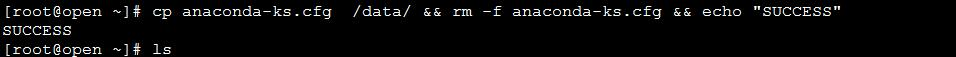|| 运算符: 格式 command1 || command2  ||则与&&相反。如果||左边的命令（command1）未执行成功，那么就执行||右边的命令（command2）；或者换句话说，“如果这个命令执行失败了||那么就执行这个命令。 命令之间使用 || 连接，实现逻辑或的功能。 只有在 || 左边的命令返回假（命令返回值$? == 1），|| 右边的命令才会被执行。这和 c 语言中的逻辑或语法功能相同，即实现短路逻辑或操作。

只要有一个命令返回真（命令返回值 $? == 0），后面的命令就不会被执行。 示例2中，如果 dir目录不存在，将输出提示信息 fail 。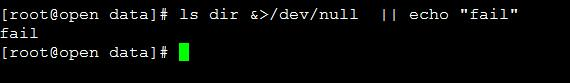示例3中，如果 dir 目录存在，将输出 success 提示信息；否则输出 fail 提示信息。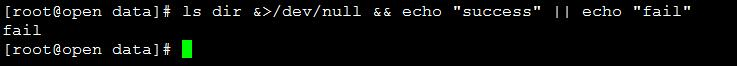6.下面是一个shell脚本中常用的||组合示例 echo$BASH |grep -q 'bash' || { exec bash "$0" "$@" || exit 1; }    系统调用exec是以新的进程去代替原来的进程，但进程的PID保持不变。因此，可以这样认为，exec系统调用并没有创建新的进程，只是替换了原来进程上下文的内容。原进程的代码段，数据段，堆栈段被新的进程所代替。


() 运算符:

如果希望把几个命令合在一起执行，shell提供了两种方法。既可以在当前shell也可以在子shell中执行一组命令。
格式:

(command1;command2;command3....)               多个命令之间用;分隔


一条命令需要独占一个物理行，如果需要将多条命令放在同一行，命令之间使用命令分隔符（;）分隔。执行的效果等同于多个独立的命令单独执行的效果。
() 表示在当前 shell 中将多个命令作为一个整体执行。需要注意的是，使用 () 括起来的命令在执行前面都不会切换当前工作目录，也就是说命令组合都是在当前工作目录下被执行的，尽管命令中有切换目录的命令。
命令组合常和命令执行控制结合起来使用。

示例4中，如果目录dir不存在，则执行命令组合。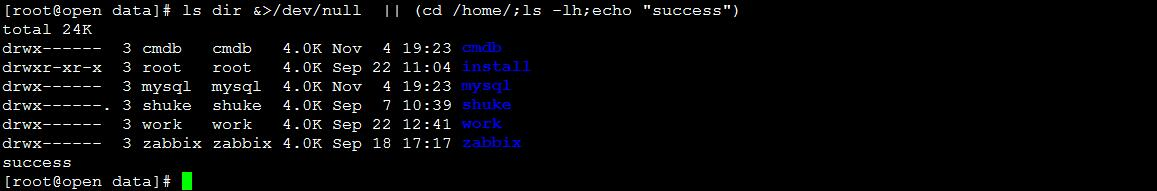{} 运算符:

如果使用{}来代替()，那么相应的命令将在子shell而不是当前shell中作为一个整体被执行，只有在{}中所有命令的输出作为一个整体被重定向时，其中的命令才被放到子shell中执行，否则在当前shell执行。
它的一般形式为：

{ command1;command2;command3… }      注意：在使用{}时，{}与命令之间必须使用一个空格


示例5中，使用{}则在子shell中执行了打印操作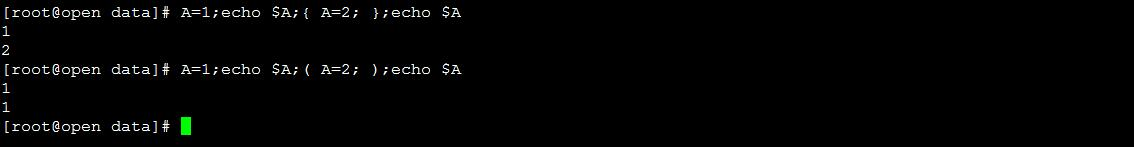展开全文• Shell运算符的分类 一，算数运算符 expr是一款表达式计算工具，使用它就能完成表达式的求值操作。 ①两个数相加,使用的是反引号 ，而不是单引号,在左上角的Esc键下方 ②expr后面的数字和符号都要空格（例如...Linux Shell脚本
• 1．基本语法 （1）“$((运算式))”或...注意：expr运算符间要有空格 2．案例实操： （1）计算3+2的值 [zj@hadoop101 datas]$ expr 2 + 3 5 （2）计算3-2的值 （a）expr一步完成计算 [zj@hadoop101 datas]$... • ## shell三目运算符 千次阅读 2019-07-02 17:30:56$(())表达式还有一个用途，三目运算： # 如果条件为真返回1，否则返回0 # echo $((1<0)) 0 # echo$((1>0)) 1 指定输出数字： # echo $((1>0?1:2)) 1 # echo$((1<0?1:2)) 2 注意：返回值不支持字符......
• 对于三目运算符大家一般都很熟悉，java中的写法是 a==b?c:d 当a==b时为c否则为d 在linux bash中用 “:-”来表示，如：export HADOOP_HOME=${HADOOP_HOME:-$LIB_DIR/hadoop} 我们在hadoop的相关脚本中容易看到这句...
• 三元运算符 ？ echo "显示启动日志" Tomcat_Home=/home/bian/tomcat-bms/ parm=$2 timeout$((parm>0?parm:5)) tail -f $Tomcat_Home/logs/catalina.out.date +%Y-%m-%d exit 0 根据传入的第2个参数n，如果n...shell三元运算符 • Shell 中位运算符的应用 位运算符通常用于整数间运算，位运算符是针对整数在内存中二进制数据流中的位进行操作。 例如：表达式“2>>1”表示将整数的二进制数2在内存中的二进制数据流向左移动一位。 ...shell • shell中的比较运算符： -eq //等于 -ne //不等于 -gt //大于 （greater） -lt //小于（less） -ge //大于等于 -le //小于等于 命令的逻辑关系： 在 linux 中命令执行状态：0 为真，其他为假 。$? 是显示最后...
• ## shell教程

千次阅读 2018-12-21 18:41:41
五、Shell运算符：Shell算数运算符、关系运算符、布尔运算符、字符串运算符等 六、shell注释 七、shell字符串 八、Shell数组：shell数组的定义、数组长度 九、Shell echo命令 十、shell p...shell
• 变量本质上存储数据的一个或多个计算机内存地址，分为本地变量（用户当前shell生命期使用，随shell进程的消亡而无效，类似局部变量）、环境变量（适用于所有由登录进程所产生的子进程）和位置参数（向shell脚本传递...
• 运算符是对计算机发的指令 运算符对象 数字、字符（子面值） 变量 表达式 表达式 运算符和运输对象的组合体。 按位运算符 * ~op1 反运算符 * op1左移运算符 * op1>>op2 右移运算符 * op1 & op2 与比较运算符 ...shell
• 1、数值 格式： test "num1" opr "num2" [ "num1" opr "num2" ] opr 取值：  相等：-eq 不等：-ne 大于：-gt 小于：-lt 【l是字母L的...大于等于：-geshell file
• 常用判断条件 -z &quot;$str&quot; #判断字符串为空 -f /home/builder/.profile #判断文件是否存在 ... -f /home/builder/.bash_profile # 逻辑非在语句前加“!...1.关系运算符 2.布尔运算 3.逻辑运...shell • 1、本地变量：在用户现在的shell声明周期的脚本中使用$ localtest="test"$echo${localtest}test$readonly localtest$ locatest="tests"bash: localtest: 只读变量$set$ readonly2、环境变量：用于所有用户进程...shell linux bash path
• Linux Shell 比较运算符 运算符 描述 示例 文件比较运算符 -e filename 如果 filename 存在，则为真 [ -e /var/log/syslog ] -d filename 如果 filename 为目录，则为真 [ -d /tmp/mydir ] ...Linux shell 比较运算符
• ## Shell浮点数运算

千次阅读 2011-05-03 22:27:00
Shell本身不支持浮点数运算，(())之类的数学运算符只能进行整数运算。要想在shell中运算浮点数，只能借助于bc, Awk或者Perl。 如 a=0.1 b=0.2 c=$(echo "$a+\$b" | bc -l)  或者 perl -e ...shell perl c
...